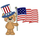9696瀏覽

Continuing deeper and stronger with the screeners' educational serie one more time

I - Concept

This is the first flexible screener I'm releasing. Screener detecting a convergence whenever the MACD and MM cross are giving a signal in the same direction.
Those who know me from TradingView ... are aware that I'm big on convergences. I totally think that 1 indicator isn't enough - whatever the timeframe.
But building my own convergence detection systems has been fruitful for me

II - How did I set the screener

The visual signals are as follow:

- square: MACD + MA cross convergence.
- diamond: Only MACD is selected
- circle: Only MA cross is selected

Then the colors are:

- green when bullish
- red when bearish

Example

Below, I highlighted why we see diamonds on the top screener panel. This is because I only selected the MACD filter

Cool Hacks

Don't forget that you can add the same indicator multiple times on your chart :)

Wishing you all the BEST trading
Dave

💰 Results/Blogs: https://bit.ly/2ULUtjo

👉 Get a \$30 discount on TradingView: https://bit.ly/2UM5OA0

## 評論teamtea92
The filters can be updated and I see a MACD filter in thereThis is quite fabulous, one of the best things on TV. Just been thru some forex pairs from yesterday and you can make hundreds of pips a day with the right settings.

Top man 👍❤How can I change Heatmaps colors to tiny squares?

heatmap_color(cond1, cond2) =>
cond1 ? color.new(color.green, 20) : cond2 ? color.new(color.red, 20) : na

lapos_x = timenow + round(change(time)*3)
lapos_y = highest(5)// + (0.15 * highest(20))

f_draw_label(x,y,_text,_textcolor, _size)=>
var label Label = na
label.delete(Label)
Label := label.new(x, y, _text, color=color.new(color.white, 20), textcolor=_textcolor, style=label.style_none, yloc=yloc.price, xloc=xloc.bar_time, size=_size)

res_to_string(res)=>
str = iff(res == "1", "m1", iff(res == "5", "m5", iff(res == "15", "m15", iff(res == "30", "m30", iff(res == "60", "1H", iff(res == "120", "H2",
iff(res == "240", "4H", iff(res == "720", "12H", iff(res == "D", "1D", iff(res == "W", "1W", iff(res == "M", "1M", "")))))))))))
str

//l1 = hline(min + 0 * incr - 0.5 * incr, color=color(#000000, 100), linewidth = 0, linestyle = dotted)
h0 = hline(1, color=color.new(color.black, 10), linewidth=2, linestyle=hline.style_solid)
h1 = hline(2, color=color.new(color.black, 10), linewidth=2, linestyle=hline.style_solid)
h2 = hline(3, color=color.new(color.black, 10), linewidth=2, linestyle=hline.style_solid)
h3 = hline(4, color=color.new(color.black, 10), linewidth=2, linestyle=hline.style_solid)
h4 = hline(5, color=color.new(color.black, 10), linewidth=2, linestyle=hline.style_solid)
h5 = hline(6, color=color.new(color.black, 10), linewidth=2, linestyle=hline.style_solid)
h6 = hline(7, color=color.new(color.black, 10), linewidth=2, linestyle=hline.style_solid)

//res1 = input("15", type=input.resolution, title="First Timeframe")
//res2 = input("60", type=input.resolution, title="Second Timeframe")
//res3 = input("240", type=input.resolution, title="Third Timeframe")
//res4 = input("720", type=input.resolution, title="Fourth Timeframe")
//res5 = input("D", type=input.resolution, title="Fifth Timeframe")
//res6 = input("W", type=input.resolution, title="Fifth Timeframe")
//res7 = input("M", type=input.resolution, title="Sixth Timeframe")

// draw the TF labels
f_draw_label(lapos_x, 1, res_to_string(res2), color.black, size.large)
f_draw_label(lapos_x, 2, res_to_string(res3), color.black, size.large)
f_draw_label(lapos_x, 3, res_to_string(res4), color.black, size.large)
f_draw_label(lapos_x, 4, res_to_string(res5), color.black, size.large)
f_draw_label(lapos_x, 5, res_to_string(res6), color.black, size.large)
f_draw_label(lapos_x, 6, res_to_string(res7), color.black, size.large)

fill(h0 ,h1, color=heatmap_color(close >= hdSAR, close <= hdSAR))
fill(h1, h2, color=heatmap_color(close >= h4SAR, close <= h4SAR))
fill(h2, h3, color=heatmap_color(close >= htSAR, close <= htSAR))
fill(h3, h4, color=heatmap_color(close >= dSAR, close <= dSAR))
fill(h4, h5, color=heatmap_color(close >= wSAR, close <= wSAR))
fill(h5, h6, color=heatmap_color(close >= mSAR, close <= mSAR))Agent87
@Agent87, for tiny square, you have to use the plotshape function with style=shape.square as I did on the script just above